# 3.6 Absolute value functions  (Page 3/3)

 Page 3 / 3

Access these online resources for additional instruction and practice with absolute value.

## Key concepts

• Applied problems, such as ranges of possible values, can also be solved using the absolute value function. See [link] .
• The graph of the absolute value function resembles a letter V. It has a corner point at which the graph changes direction. See [link] .
• In an absolute value equation, an unknown variable is the input of an absolute value function.
• If the absolute value of an expression is set equal to a positive number, expect two solutions for the unknown variable. See [link] .

## Verbal

How do you solve an absolute value equation?

Isolate the absolute value term so that the equation is of the form $\text{\hspace{0.17em}}|A|=B.\text{\hspace{0.17em}}$ Form one equation by setting the expression inside the absolute value symbol, $\text{\hspace{0.17em}}A,\text{\hspace{0.17em}}$ equal to the expression on the other side of the equation, $\text{\hspace{0.17em}}B.\text{\hspace{0.17em}}$ Form a second equation by setting $\text{\hspace{0.17em}}A\text{\hspace{0.17em}}$ equal to the opposite of the expression on the other side of the equation, $\text{\hspace{0.17em}}-B.\text{\hspace{0.17em}}$ Solve each equation for the variable.

How can you tell whether an absolute value function has two x -intercepts without graphing the function?

When solving an absolute value function, the isolated absolute value term is equal to a negative number. What does that tell you about the graph of the absolute value function?

The graph of the absolute value function does not cross the $\text{\hspace{0.17em}}x$ -axis, so the graph is either completely above or completely below the $\text{\hspace{0.17em}}x$ -axis.

How can you use the graph of an absolute value function to determine the x -values for which the function values are negative?

## Algebraic

Describe all numbers $\text{\hspace{0.17em}}x\text{\hspace{0.17em}}$ that are at a distance of 4 from the number 8. Express this set of numbers using absolute value notation.

Describe all numbers $\text{\hspace{0.17em}}x\text{\hspace{0.17em}}$ that are at a distance of $\text{\hspace{0.17em}}\frac{1}{2}\text{\hspace{0.17em}}$ from the number −4. Express this set of numbers using absolute value notation.

$\text{\hspace{0.17em}}|x+4|=\frac{1}{2}\text{\hspace{0.17em}}$

Describe the situation in which the distance that point $\text{\hspace{0.17em}}x\text{\hspace{0.17em}}$ is from 10 is at least 15 units. Express this set of numbers using absolute value notation.

Find all function values $\text{\hspace{0.17em}}f\left(x\right)\text{\hspace{0.17em}}$ such that the distance from $\text{\hspace{0.17em}}f\left(x\right)\text{\hspace{0.17em}}$ to the value 8 is less than 0.03 units. Express this set of numbers using absolute value notation.

$|f\left(x\right)-8|<0.03$

For the following exercises, find the x - and y -intercepts of the graphs of each function.

$f\left(x\right)=4|x-3|+4$

$f\left(x\right)=-3|x-2|-1$

$\left(0,-7\right);\text{\hspace{0.17em}}$ no $\text{\hspace{0.17em}}x$ -intercepts

$f\left(x\right)=-2|x+1|+6$

$f\left(x\right)=-5|x+2|+15$

$\left(0,\text{\hspace{0.17em}}5\right),\left(1,0\right),\left(-5,0\right)$

$f\left(x\right)=2|x-1|-6$

$\left(0,-4\right),\left(4,0\right),\left(-2,0\right)$

$f\left(x\right)=|-2x+1|-13$

$\left(0,-12\right),\left(-6,0\right),\left(7,0\right)$

$f\left(x\right)=-|x-9|+16$

$\left(0,7\right),\left(25,0\right),\left(-7,0\right)$

## Graphical

For the following exercises, graph the absolute value function. Plot at least five points by hand for each graph.

$y=|x-1|$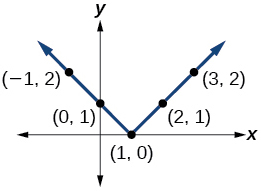$y=|x+1|$

$y=|x|+1$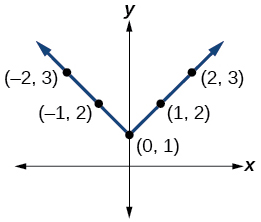For the following exercises, graph the given functions by hand.

$y=|x|-2$

$y=-|x|$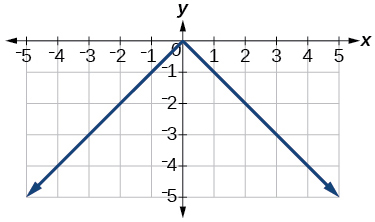$y=-|x|-2$

$y=-|x-3|-2$$f\left(x\right)=-|x-1|-2$

$f\left(x\right)=-|x+3|+4$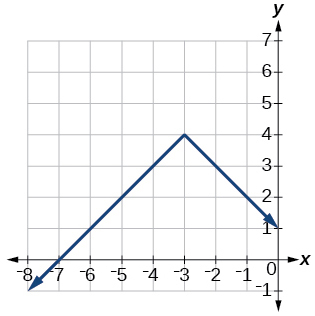$f\left(x\right)=2|x+3|+1$

$f\left(x\right)=3|x-2|+3$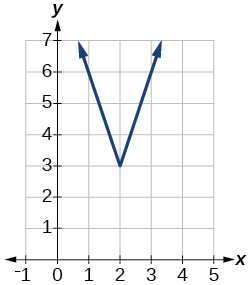$f\left(x\right)=|2x-4|-3$

$f\left(x\right)=|3x+9|+2$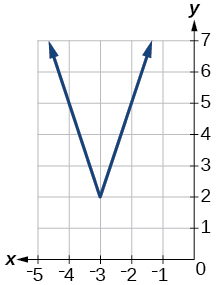$f\left(x\right)=-|x-1|-3$

$f\left(x\right)=-|x+4|-3$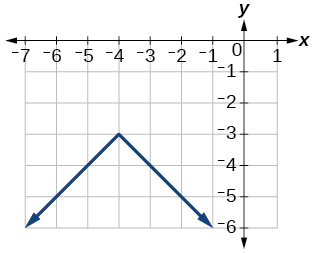$f\left(x\right)=\frac{1}{2}|x+4|-3$

## Technology

Use a graphing utility to graph $f\left(x\right)=10|x-2|$ on the viewing window $\left[0,4\right].$ Identify the corresponding range. Show the graph.

range: $\text{\hspace{0.17em}}\left[0,20\right]$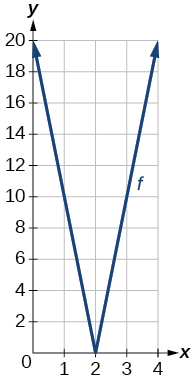Use a graphing utility to graph $\text{\hspace{0.17em}}f\left(x\right)=-100|x|+100\text{\hspace{0.17em}}$ on the viewing window $\text{\hspace{0.17em}}\left[-5,5\right].\text{\hspace{0.17em}}$ Identify the corresponding range. Show the graph.

For the following exercises, graph each function using a graphing utility. Specify the viewing window.

$f\left(x\right)=-0.1|0.1\left(0.2-x\right)|+0.3$

$x\text{-}$ intercepts: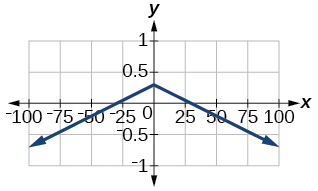$f\left(x\right)=4×{10}^{9}|x-\left(5×{10}^{9}\right)|+2×{10}^{9}$

## Extensions

For the following exercises, solve the inequality.

If possible, find all values of $a$ such that there are no $x\text{-}$ intercepts for $f\left(x\right)=2|x+1|+a.$

If possible, find all values of $\text{\hspace{0.17em}}a\text{\hspace{0.17em}}$ such that there are no $\text{\hspace{0.17em}}y$ -intercepts for $\text{\hspace{0.17em}}f\left(x\right)=2|x+1|+a.$

There is no solution for $\text{\hspace{0.17em}}a\text{\hspace{0.17em}}$ that will keep the function from having a $\text{\hspace{0.17em}}y$ -intercept. The absolute value function always crosses the $\text{\hspace{0.17em}}y$ -intercept when $\text{\hspace{0.17em}}x=0.$

## Real-world applications

Cities A and B are on the same east-west line. Assume that city A is located at the origin. If the distance from city A to city B is at least 100 miles and $\text{\hspace{0.17em}}x\text{\hspace{0.17em}}$ represents the distance from city B to city A, express this using absolute value notation.

The true proportion $\text{\hspace{0.17em}}p\text{\hspace{0.17em}}$ of people who give a favorable rating to Congress is 8% with a margin of error of 1.5%. Describe this statement using an absolute value equation.

$|p-0.08|\le 0.015$

Students who score within 18 points of the number 82 will pass a particular test. Write this statement using absolute value notation and use the variable $\text{\hspace{0.17em}}x\text{\hspace{0.17em}}$ for the score.

A machinist must produce a bearing that is within 0.01 inches of the correct diameter of 5.0 inches. Using $\text{\hspace{0.17em}}x\text{\hspace{0.17em}}$ as the diameter of the bearing, write this statement using absolute value notation.

$|x-5.0|\le 0.01$

The tolerance for a ball bearing is 0.01. If the true diameter of the bearing is to be 2.0 inches and the measured value of the diameter is $\text{\hspace{0.17em}}x\text{\hspace{0.17em}}$ inches, express the tolerance using absolute value notation.

#### Questions & Answers

The sequence is {1,-1,1-1.....} has
amit Reply
circular region of radious
Kainat Reply
how can we solve this problem
Joel Reply
Sin(A+B) = sinBcosA+cosBsinA
Eseka Reply
Prove it
Eseka
Please prove it
Eseka
hi
Joel
June needs 45 gallons of punch. 2 different coolers. Bigger cooler is 5 times as large as smaller cooler. How many gallons in each cooler?
Arleathia Reply
find the sum of 28th term of the AP 3+10+17+---------
Prince Reply
I think you should say "28 terms" instead of "28th term"
Vedant
if sequence sn is a such that sn>0 for all n and lim sn=0than prove that lim (s1 s2............ sn) ke hole power n =n
SANDESH Reply
write down the polynomial function with root 1/3,2,-3 with solution
Gift Reply
if A and B are subspaces of V prove that (A+B)/B=A/(A-B)
Pream Reply
write down the value of each of the following in surd form a)cos(-65°) b)sin(-180°)c)tan(225°)d)tan(135°)
Oroke Reply
Prove that (sinA/1-cosA - 1-cosA/sinA) (cosA/1-sinA - 1-sinA/cosA) = 4
kiruba Reply
what is the answer to dividing negative index
Morosi Reply
In a triangle ABC prove that. (b+c)cosA+(c+a)cosB+(a+b)cisC=a+b+c.
Shivam Reply
give me the waec 2019 questions
Aaron Reply
the polar co-ordinate of the point (-1, -1)
Sumit Reply

### Read also:

#### Get the best Algebra and trigonometry course in your pocket!

Source:  OpenStax, Algebra and trigonometry. OpenStax CNX. Nov 14, 2016 Download for free at https://legacy.cnx.org/content/col11758/1.6
Google Play and the Google Play logo are trademarks of Google Inc.

Notification Switch

Would you like to follow the 'Algebra and trigonometry' conversation and receive update notifications?ByByByByBy